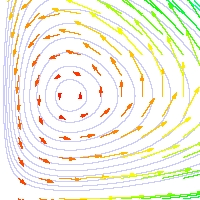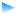Spring 2004

# Mathematics Math1b Spring 2004

## Introduction to Functions and Calculus II and Differential equations

Office: SciCtr 429
Email: gottlieb@math.harvard.eduMainpage Syllabus Sections Calendar Homework Exams Supplements Links

## Exams

We will use this page to post old exams and solutions to help you study and to post reveiw problems and the solutions.The Integration Technique Test. Here is a list of integral problems and answers from which at least 70% of the technique test questions will be chosen.The first midterm

Here are some previous exams and solutions that should provide a good review of the material you have covered to date.

 Fall 2001 (Questions 2,3,5,7, and 8 only) Solutions Spring 2000 (Questions 2,5,6, and 7c only) Solutions Spring 1999 (Questions 1,2,3, and 4 only) Solutions Fall 1998 (Questions 5,6 and 7 only) Solutions Review Problem Sheet Soltutions

There is also a REVIEW SHEET for the first exam now posted under Supplements.

Finally, below you can find the solutions to this year's first midterm:The second midterm

Here are some previous exams and solutions that should provide a good review of the material you have covered to date. Because of changes in the syllabus, you are not responsible for problems that involve the Taylor remainder theorem.These problems are noted below:

 Spring 1999 Solutions Fall 1999 Solutions Fall 1998 Solutions Review Problem Sheet (Neglect 25c) Soltutions

Here is a review sheet prepared for the second midterm.

Review Sheet
(Neglect page 7)

Finally, below you can find the solutions to this year's first midterm:Final

Past final exams can be found below. You are not responsible for problems requiring the Taylor Remainder, Surface Area, and solving differential equations with series.

 Fall 1995 Solutions Fall 1998 (Omit 3a) Solutions Fall 1999 Solutions In #8: the differ'l eqn is BOTH first order linear AND separable. The answer is y = Ce^(x^2/2) - 1. (The exponent got cut off.) Spring 1999 (Omit 2b) Also, note that in 3, you could have simply been asked for the solution. Soltutions

Here is a differential equation review sheet.

And a more qualitative one.

(Note: Part of #8 is mysteriously absent. The equation is x'' +x' + 4x =0 and the initial conditions are x(0) = -1 and x'(0) = -1.)